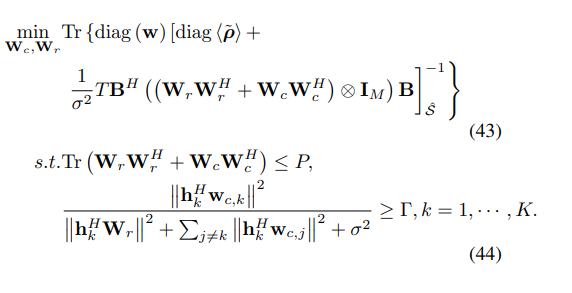# SINR Constraints don't work

My original problem is as follows:And my code is as follows

" w_diag = zeros(N_point,1);

``````for i = 1:L
w_diag(index_xr(i)) = sqrt(w(i));
end

sigma_comm = 0;

cvx_begin

variable Wr(M,M) complex  % the dimension of radar beamforing matrix: M*M
variable Wc(M,M) complex  % the dimension of comm beamforing matrix

MSE = (diag(rou)+(1/(sigma^2))*T*B'*kron((Wr+Wc),eye(M))*B);
% rou,B are known already which means they're certain vectors, and "sigma,T,M" are scalars.
MSE_inv = matrix_frac(w_diag,MSE);

minimize real(MSE_inv)

subject to

real(eta*h*Wr*h') <= real(h*Wc*h') ;
real(trace(Wc)+trace(Wr)) <= P;
Wr == hermitian_semidefinite(M)
Wc == hermitian_semidefinite(M)

cvx_end"
``````

My target function is to minimize the performance of radar/sensing, so i think the constraints of communication, i.e. "real(etahWrh’) <= real(hWc*h’) " would contradict with it. However when i run the code and get the answer, the constraint term does not take equal signs. And usually the right-hand side is twice the left-hand side. Can someone help me figure out what the problem might be?

when I set P=1, cvx outputs failed and when I enlarge P to 20, solver outputs inaccurated solved. but the constraints are still not satisfied.

## Calling SDPT3 4.0: 1098 variables, 334 equality constraints For improved efficiency, SDPT3 is solving the dual problem.

num. of constraints = 334
dim. of sdp var = 61, num. of sdp blk = 3
dim. of linear var = 2
dim. of free var = 665 *** convert ublk to lblk

SDPT3: Infeasible path-following algorithms

## number of iterations = 17 primal objective value = -9.40900341e-03 dual objective value = -8.91968790e-03 gap := trace(XZ) = 4.26e-06 relative gap = 4.18e-06 actual relative gap = -4.81e-04 rel. primal infeas (scaled problem) = 3.72e-05 rel. dual " " " = 2.60e-09 rel. primal infeas (unscaled problem) = 0.00e+00 rel. dual " " " = 0.00e+00 norm(X), norm(y), norm(Z) = 1.0e+00, 1.3e+03, 1.9e+04 norm(A), norm(b), norm© = 1.9e+04, 2.0e+00, 6.4e+03 Total CPU time (secs) = 0.86 CPU time per iteration = 0.05 termination code = -5 DIMACS: 3.7e-05 0.0e+00 7.1e-09 0.0e+00 -4.8e-04 4.2e-06

Status: Inaccurate/Solved
Optimal value (cvx_optval): +0.00891969

i just wondering why the result always be the twice relationship for the communication SINR constraints?by the way I have ignored the noise of communication in my problem.

Can you please consolidate all your posts on one problem into one topic (question)? if the posts in this topic are still relevant, please post whatever is still relevant in How to formulate the target function that cvx can solve

As it is, it is too difficult to figure out which posts supersede other posts, if at all.

And you can’t expect readers of this forum to be experts in your application area. it is incumbent on you to properly formulate a convex optimization problem which adequately models your intended application.